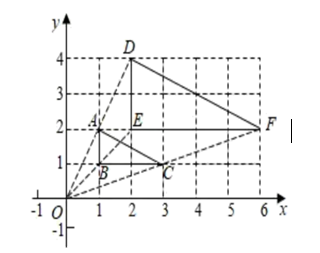$\text{A.}$ $\sqrt{5}$ $\text{B.}$ $2$ $\text{C.}$ $4$ $\text{D.}$ $2 \sqrt{5}$

D

#### 解析：

\begin{aligned} & \therefore \mathrm{D}(2,4), \mathrm{F}(6,2), \\ & \therefore \mathrm{DF}=\sqrt{(2-6)^2+(4-2)^2}=2 \sqrt{5}, \end{aligned}

①点击 首页查看更多试卷和试题 , 点击查看 本题所在试卷